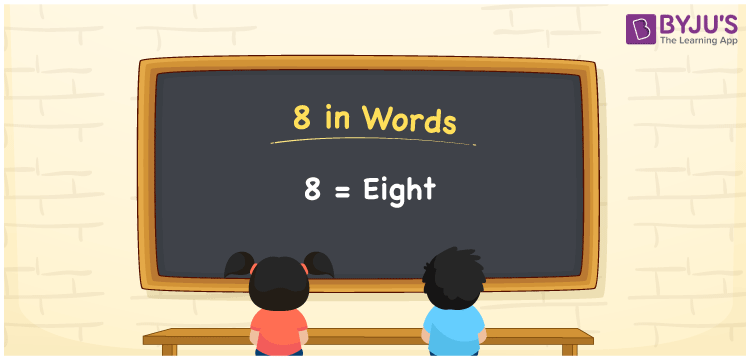# 8 in Words

8 in words is written as Eight. In both the International System of Numerals and the Indian System of Numerals, 8 in words is written as Eight. The number 8 is a Cardinal Number as it could represent some quantity. For example, “there are 8 notebooks in that bag”.

 8 in Words Eight Eight in Number 8

## 8 in English Words

We write 8 in English Words using the English alphabet letters. Therefore, we spell 8 in English as “Eight”.## How to Write 8 in Words?

To write 8 in words, we shall use the place value chart. In the place value chart, write 8 in the ones place and 0 in all the rest. Let us make a place value chart to write the number 8 in words.

 Hundreds Tens Ones 0 0 8

Thus, we can write the expanded form as

0 × Hundred + 0 × Ten + 8 × One

= 0 × 100 + 0 × 10 + 8 × 1

= 0 + 0 + 8

= 8

= Eight

8 is the 8th natural number that lies between 7 and 9.

8 in words – Eight

• Is 8 an odd number? – No
• Is 8 an even number? – Yes
• Is 8 a perfect square number? – No
• Is 8 a perfect cube number? – Yes; 23 = 8
• Is 8 a prime number? – No
• Is 8 a composite number? – Yes

## Frequently Asked Questions on 8 in Words

### How to write 8 in English words?

8 in words is written as Eight.

### How to spell 8?

The spelling of 8 is “Eight.”

### Is 8 a Whole Number?

Yes, 8 is a Whole Number.